# Forced Convective Heat Transfer (Part - 5) Chemical Engineering Notes | EduRev

## Chemical Engineering : Forced Convective Heat Transfer (Part - 5) Chemical Engineering Notes | EduRev

The document Forced Convective Heat Transfer (Part - 5) Chemical Engineering Notes | EduRev is a part of the Chemical Engineering Course Heat Transfer for Engg..
All you need of Chemical Engineering at this link: Chemical Engineering

4.4 Momentum and heat transfer analogies

Consider a fluid flows in a circular pipe in a laminar low (fig.6.6). The wall of the pipe is maintained at Tw temperature, which is higher than the flowing fluid temperature. The fluid being in relatively lower temperature than the wall temperature will get heated as it flows through the pipe. Moreover, the radial transport of the momentum in the pipe occurs as per the Newton’s law of viscosity.  For a circular pipe momentum transport and heat transport may be written in a similar way as shown in the eq. 4.28,

Momentum flux = momentum diffusivity × gradient of concentration of momentum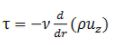(4.28 (a))

It may be noted that the fluid velocity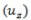is a function of radius of the pipe.

Heat flux= thermal diffusivity × gradient of concentration of heat energy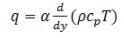(4.28 (b))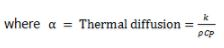Now, the question comes, why are we discussion about the similarities? The answer is straight forward that it is comparatively easy to experimentally/theoretically evaluate the momentum transport under various conditions. However, the heat transport is not so easy to find out. Therefore, we will learn different analogies to find the heat transport relations.

Equation 4.28 is for the laminar flow but if the flow is turbulent, eddies are generated. Eddy is a lump/chunk of fluid elements that move together. Thus it may be assumed that the eddies are the molecules of the fluid and are responsible for the transport of momentum and heat energy in the turbulent flow. Therefore, in turbulent situation the momentum and heat transport is not only by the molecular diffusion but also by the eddy diffusivities.

Thus, turbulent transport of momentum and turbulent transport of heat may be represented by eq. 4.29a and 4.29b, respectively.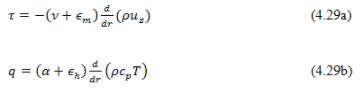The terms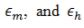represent the eddy diffusivities for momentum and heat, respectively.
At the wall of the pipe, the momentum equation (eq. 4.29a) becomes,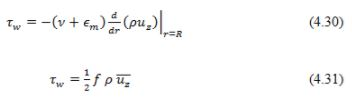Where f is the fanning friction factor (ratio of shear force to inertial force) and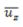is the average fluid velocity.

Equation eq.4.30 can be rearranged as,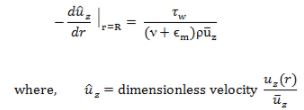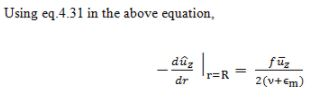(4.32)

The eq. 4.32 is the dimensionless velocity gradient at the wall using momentum transport. We may get the similar relation using heat transport as shown below.
Wall heat flux can be written as,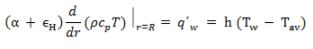Where Tav is the wall temperature and the Tav is the average temperature of the fluid. Thus, the dimensionless temperature gradient at the wall using heat transfer will be,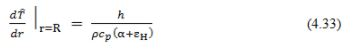Where the heat transfer coefficient is represented by h and dimensionless temperature is represented by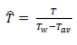.

Based on the above discussion many researchers have given their analogies. These analogies are represented in the subsequent section

Offer running on EduRev: Apply code STAYHOME200 to get INR 200 off on our premium plan EduRev Infinity!

## Heat Transfer for Engg.

46 videos|60 docs|59 tests

,

,

,

,

,

,

,

,

,

,

,

,

,

,

,

,

,

,

,

,

,

;